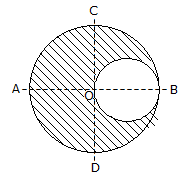Courses

# Test: Engineering Mechanics - 7

## 25 Questions MCQ Test Engineering Mechanics | Test: Engineering Mechanics - 7

Description
This mock test of Test: Engineering Mechanics - 7 for Mechanical Engineering helps you for every Mechanical Engineering entrance exam. This contains 25 Multiple Choice Questions for Mechanical Engineering Test: Engineering Mechanics - 7 (mcq) to study with solutions a complete question bank. The solved questions answers in this Test: Engineering Mechanics - 7 quiz give you a good mix of easy questions and tough questions. Mechanical Engineering students definitely take this Test: Engineering Mechanics - 7 exercise for a better result in the exam. You can find other Test: Engineering Mechanics - 7 extra questions, long questions & short questions for Mechanical Engineering on EduRev as well by searching above.
QUESTION: 1

Solution:
QUESTION: 2

Solution:
QUESTION: 3

### Efficiency of a screw jack is given by(where α = Helix angle, and φ = Angle of friction.)

Solution:
QUESTION: 4

When a train is rounding a curve, the side thrust on the wheel flanges is prevented by raising the outer edge of the rail.

Solution:
QUESTION: 5

Frequency of vibrations means the number of cycles per second.

Solution:
QUESTION: 6

The time of flight (t) of a projectile on a horizontal plane is given by

Solution:
QUESTION: 7

The velocity ratio for the third system of pulleys is

Solution:
QUESTION: 8

The moment of inertia of a thin spherical shell of mass m and radius r, about its diameter is

Solution:
QUESTION: 9

If the gravitational acceleration at any place is doubled, then the weight of a body will be

Solution:

Correct Answer :- d

Explanation : Weight = density * acceleration

If the gravitational acceleration at any place is doubled, then the weight of a body will be doubled.

QUESTION: 10

The resultant of two forces P and Q (such that P > Q) acting along the same straight line, but in opposite direction, is given by

Solution:
QUESTION: 11

The velocity ratio for the second system of pulleys is n.

Solution:
QUESTION: 12

The law of motion involved in the recoil of gun is

Solution:
QUESTION: 13

When a particle moves along a circular path, its acceleration has two components, one is normal component and the other is tangential component of acceleration.

Solution:
QUESTION: 14

A machine which is not capable of doing any work in the reversed direction, after the effort is removed, is called a reversible machine.

Solution:
QUESTION: 15

The range of projectile will be maximum for a given velocity of projectile, when the angle of projection (α) is

Solution:
QUESTION: 16

When two elastic bodies collide with each other,

Solution:
QUESTION: 17

In order to double the period of simple pendulum, the length of the string should be

Solution:
QUESTION: 18

The centre of gravity of a triangle lies at a point where its medians intersect each other.

Solution:
QUESTION: 19

The moment of inertia of a square of side a about its base is a4/ 3.

Solution:
QUESTION: 20

If the number of pulleys in a system is equal to its velocity ratio, then it is a __________ system of pulleys

Solution:
QUESTION: 21

The centre of gravity of a right angled triangle lies at its geometrical centre.

Solution:
QUESTION: 22

Vectors method for the resultant force is also called polygon law of forces.

Solution:
QUESTION: 23

Two equal and opposite parallel forces whose lines of action are different form a couple.

Solution:
QUESTION: 24

When a person, on a bicycle, drives round a curve, he has to lean __________ to maintain equilibrium.

Solution:
QUESTION: 25

A circular hole of 50 mm diameter is cut out from a circular disc of 100 mm diameter as shown in the below figure. The centre of gravity of the section will lieSolution: